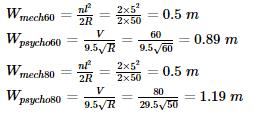Courses

# Transportation Engineering 1

## 10 Questions MCQ Test RRB JE for Civil Engineering | Transportation Engineering 1

Description
This mock test of Transportation Engineering 1 for Civil Engineering (CE) helps you for every Civil Engineering (CE) entrance exam. This contains 10 Multiple Choice Questions for Civil Engineering (CE) Transportation Engineering 1 (mcq) to study with solutions a complete question bank. The solved questions answers in this Transportation Engineering 1 quiz give you a good mix of easy questions and tough questions. Civil Engineering (CE) students definitely take this Transportation Engineering 1 exercise for a better result in the exam. You can find other Transportation Engineering 1 extra questions, long questions & short questions for Civil Engineering (CE) on EduRev as well by searching above.
QUESTION: 1

### A super-elevation e is provided on a circular horizontal curve such that a vehicle can be stopped on the curve without sliding. Assuming a design speed v and maximum coefficient of side friction fmax, which one of the following criteria should be satisfied?

Solution: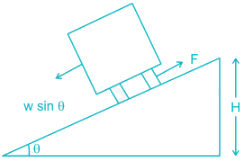Let the weight of vehicle = W in stopping condition the friction force F

should be greater than w sin θ to prevent the sliding F > w sin θ

for smaller value of Q

tan θ = e = sin θ

F = w × f

w × f ≥ w × e

f > e

QUESTION: 2

### Which of the following statements CANNOT be used to describe free-flow speed (uf) of a traffic stream?

Solution:

Free flow speed (Vf) is that speed at which flow and density is negligible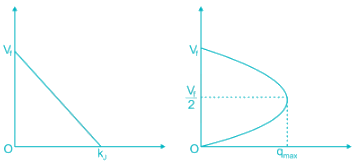QUESTION: 3

### The safety within a roundabout and the efficiency of a roundabout can be increased respectively by

Solution:

The essence of the intersection control is to resolve these conflicts at the intersection for the safe and efficient movement of both vehicular traffic and pedestrians.

The safety within a roundabout and the efficiency of a roundabout can be increased respectively by decreasing the entry radius and increasing the exit radius.

Note:

There are two methods of intersection controls

1) time sharing and

2) space sharing.

The type of intersection control that has to be adopted depends on the traffic volume, road geometry, cost involved, importance of the road etc.

*Answer can only contain numeric values
QUESTION: 4

While aligning a hill road with a ruling gradient of 6%, a horizontal curve of radius 50 m is encountered. The grade compensation (in percentage up two equal places) to be provided for this case would be _______

Solution:

R = 50

Grade compensation =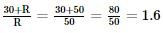(Grade compensation)max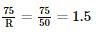Hence, (grade compensation)max = 1.5

QUESTION: 5

If the total number of commercial vehicles per day ranges from 3000 to 6000, the minimum percentage of commercial traffic to be surveyed for axle load is:

Solution:

Minimum percentage is 15% for axle load determination when commercial vehicles vary from 3000-6000.

Sample size for axle load survey: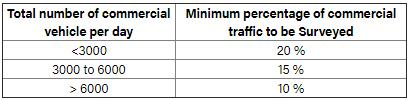*Answer can only contain numeric values
QUESTION: 6

The VPI (vertical point of intersection) is 100 m away (when measured along the horizontal) from the VPC (vertical point of curvature). If the vertical curve is parabolic the length of the curve (in meters and measured along the horizontal) is ________

Solution: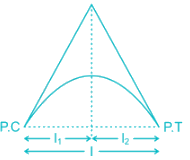For flat vertical curve we assume that l= l2

l = l1 + l2 = 100 + 100 = 200 m

QUESTION: 7

A circular curve of radius R connects two straights with a deflection angle of 60°. The tangent length is

Solution: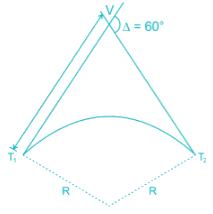Tangent length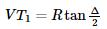= R tan 30° = 0.577 R

QUESTION: 8

On a circular curve, the rate of super-elevation is e. While negotiating the curve a vehicle comes to a stop. It is seen that the stopped vehicle does not slide inwards (in the radial direction). The coefficient of side friction is f. Which of the following is true:

Solution: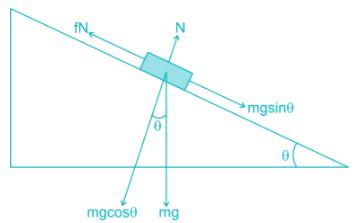For equilibrium

fN ≥ mg sin θ

⇒ fmg cos θ ≥ mg sin θ

⇒ fcos θ ≥ sin θ

⇒ f ≥ tan θ

f ≥ e

*Answer can only contain numeric values
QUESTION: 9

In a one-lane one-way homogeneous traffic stream, the observed average headway is 3.0 s. The flow (expressed in vehicles/hr) in this traffic stream is________

Solution:

Average headway= 3.0 sec

Flow of traffic stream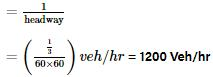QUESTION: 10

A two-lane, one-way road with a radius of 50 m is predominantly carrying lorries with a wheelbase of 5 m. The speed of lorries is restricted to be between 60 kmph and 80 kmph. The mechanical widening and psychological widening required at 60 kmph are designated as wme,60 and wps,60, respectively. The mechanical widening and psychological widening required at 80 kmph are designated as wme,80 and wps,80, respectively. The correct values of wme,60, wps,60, wme,80, wps,80, respectively are

Solution: